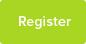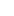# Tutor Hunt Questions

why does E=mc2??
3 years ago

Please enter your response to the question below. The student will get a notification as soon your response has been approved by our moderation team.

There is no intuitive answer, however consider energy as distance times force E=DF.

Force as you know is mass x acceleration so then (E=DMA).

You also know that acceleration is velocity divided by time which is the same as distance over time squared
(E = DMD/T2 = MD2/T2).

So energy has units of mass times velocity squared.

Now Physicists like constants.
Can you think of a UNIVERSAL relationship between mass and energy? then it should depend on universal constants.
Can you think of a universal speed?
E = mc2

c2 is the square of the speed of light.

Answered by Kevin | 3 years ago
This is the Energy-Mass equivalence equation proposed by Einstein. E is the energy here, which can be converted into mass,m, through above relation. c is the speed of light in vacuum.
two reasons:

1. The duality nature of light.
2. The conservation of total energy such that workdone = P.E + K.E = 2 KE

The duality nature of light allows us to obtain the kinetic energy of a photon using the mass of a group of ejected nucleons and the speed of light.

Conservation of total energy allows us to relate P.E (which although is potential energy but simply reflects the strength of the bond or electric potential in the nucleus).

Combining this, the energy obtained by breaking both bonds and ejecting photons in the form of light is thus the total energy E=mc2
This equation is a conclusion of special relativity (this deals with constant velocity reference frames i.e. non-accelerating frames. General relativity deals with accelerating reference frames.

Assumption:

The speed of light is always constant relative to any observer who is in a constant velocity reference frame ( different to Newtonian mechanics). The reference frame is a coordinate system with the observer at the origin to which all events relevant are referred.

From this assumption it is an algebraic argument to derive E = mc2
Answered by David | 3 years ago
Mass, such as a uranium atom, has a `rest energy` which has nothing to do with kinetic energy, potential energy, or anything to do with motion.

When nuclear reactions happen, mass can be converted directly into radiation, or kinetic energy. An extreme example would be nuclear bombs - the bomb which the US dropped on Hiroshima was estimated to have converted 0.91kg of uranium to energy. Small mass results in a big energy.
Answered by Finlay | 3 years ago
This Einstein equation of relativity that relates the inter conversion of mass into energy and energy into mass . C is the constant which is the speed of light. So I beleive it means that a mass can be converted into energy if it gains a velocity equal to the square of light speed. Now when you will try to convert the same energy into mass , it will not be the same as some of the energy will be dissipated as loss and hence will not give you the equal mass .
Answered by Aurang | 3 years ago
Energy = mass x the speed of light(squared).

This refers to the amount of energy held an object. The mass of the object has a lot to do with it because it tells you how heavy that object is, thus telling you how hard/easy it is for that object to move. The speed of light tells you how fast light takes to travel through space: the mass of the object multiplied by the speed of light (the fastest travelling thing, to our knowledge) will therefore tell you how much energy that it takes for that object to be moved through space, and, therefore, how much energy is in that object.
Answered by John | 3 years ago